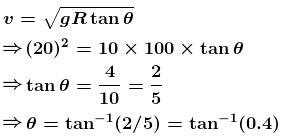Courses

# Circular Motion NAT Level - 2

## 10 Questions MCQ Test Basic Physics for IIT JAM | Circular Motion NAT Level - 2

Description
This mock test of Circular Motion NAT Level - 2 for Physics helps you for every Physics entrance exam. This contains 10 Multiple Choice Questions for Physics Circular Motion NAT Level - 2 (mcq) to study with solutions a complete question bank. The solved questions answers in this Circular Motion NAT Level - 2 quiz give you a good mix of easy questions and tough questions. Physics students definitely take this Circular Motion NAT Level - 2 exercise for a better result in the exam. You can find other Circular Motion NAT Level - 2 extra questions, long questions & short questions for Physics on EduRev as well by searching above.
*Answer can only contain numeric values
QUESTION: 1

### A ball is projected making and angle θ with the vertical. Consider a small part of the trajectory near the highest position and take it approximately to be a circular arc. What is the radius of this circle? This radius is called the radius of curvature (ROC) of the curve at the point if u = 20 then ROC  in m.

Solution: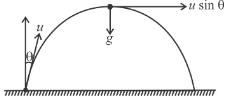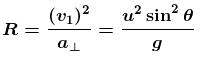*Answer can only contain numeric values
QUESTION: 2

### A small wedge whose base is horizontal is fixed to a vertical rod as shown in the figure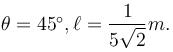The slopping side of the wedge is frictionless and the wedge is spun with a constant angular speed ω about vertical axis as shown in the figure. Find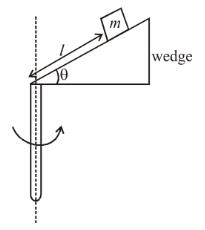The value of angular speed ω (rad/sec) for which the block of mass m just does not slide down the wedge?

Solution:

The free body diagram of the block is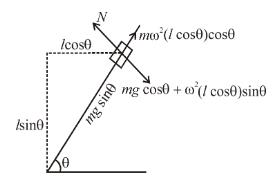For block not to slide along wedge, applying Newton's second law along incline we get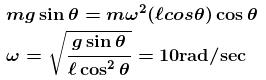*Answer can only contain numeric values
QUESTION: 3

### The member OA rotates about a horizontal axis through O with a constant counter clockwise velocity  ω = 3 rad/sec. As it passes the position θ = 0, a small mass m is placed upon it at a radial distance r = 0.5m. If the mass is observed to slip at  θ = 37°, the coefficient of friction between the mass and the member is ____ .

Solution:

As the mass is at the verge of slipping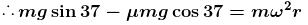6 – 8µ = 4.5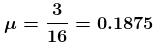*Answer can only contain numeric values
QUESTION: 4

A rod AB is moving on a fixed circle of radius R with constant velocity v’ as shown in figure. P is the point of intersection of the rod and the circle. At an instant the rod is at a distance x = 3R/5 from centre of the circle. The velocity of the rod is perpendicular to the rod and the rod is always parallel to the diameter CD.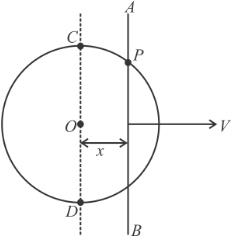The speed of point of intersection P is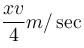then x is

Solution: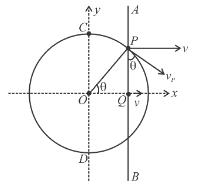As a rod AB moves, the point ‘P will always lie on the circle.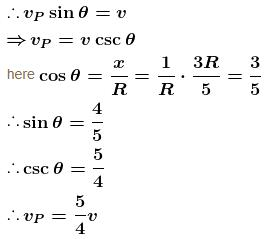x = 5

*Answer can only contain numeric values
QUESTION: 5

A squirrel climbing up a cylindrical post spirally makes the circle in a vertical height of four feet. If the top of the post is sixteen feet high and three feet in circumference, the distance travelled by it to reach the top is x in feet. Find the value of x/10

Solution:

Distance traveled in climbing 4 feet is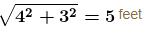So, distance traveled in climbing 16 feet will be 20 feet x = 20 feet

x/10 = 2

*Answer can only contain numeric values
QUESTION: 6

The figure shows the velocity and acceleration of a point like body at the initial moment of its motion. The acceleration vector of the body remains constant. The minimum radius of curvature of trajectory of the body is (in m.)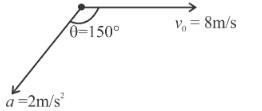Solution:

The acceleration vector shall change the component of velocity u|| along the acceleration vector.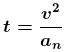Radius of curvature rmin means v is minimum and an is maximum. This is at point P when component of velocity parallel to acceleration vector becomes zero, that is u|| = 0.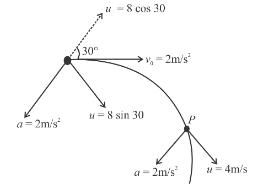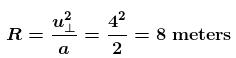*Answer can only contain numeric values
QUESTION: 7

A ring of mass 2π kg and of radius 0.25m is making 300 -rpm about an axis through its centre perpendicular to its plane. The tension (in newtons) developed in the ring is :

Solution: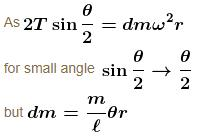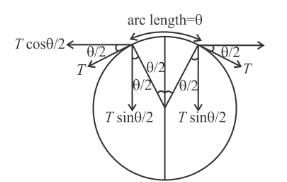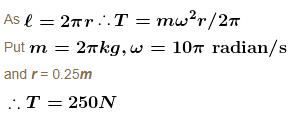*Answer can only contain numeric values
QUESTION: 8

A toy car of mass m can travel at a fixed speed. It moves in a circle on a fixed horizontal table. A string is connected to car and attached to a block of mass M = 5m that hangs as shown in figure (the portion of string below the table is always vertical). The coefficient of friction between the surface of table and tyres of the toy car is µ = 1. The ratio of the maximum radius to the minimum radius for which the toy car can move in a circular path with centre O on table is x/4, then x is: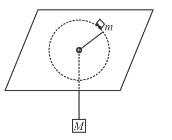Solution:

As the car travels at a fixed speed 1 m/s, hence tangential acceleration will be zero. Therefore, there will be no component of friction along tangent.

Case I : If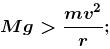hence friction force on car of mass m will be outwards from the centre.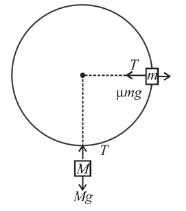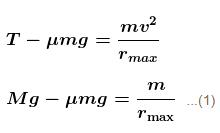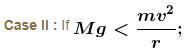hence friction force on the car of mass m will be towards centre.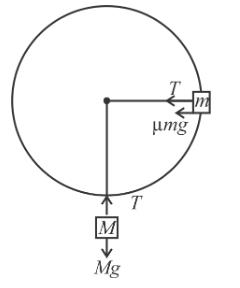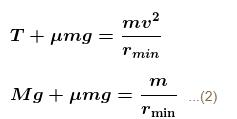From equations (1) and (2)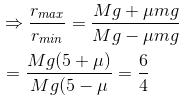*Answer can only contain numeric values
QUESTION: 9

A small bead of mass m = 1 kg is carried by a circular hoop having centre at C and radius r = 1m  which rotates about a fixed vertical axis (as shown). The coefficient of friction between bead and hoop is µ = 0.5. The maximum angular speed of the hoop for which the bead does not have relative motion with respect to hoop is given by (n√2)1/2.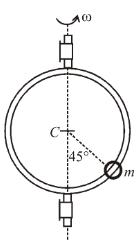Solution:

The maximum angular speed of the hoop corresponds to the situation when the bead is just about to slide upwards.
The free body diagram of the beads is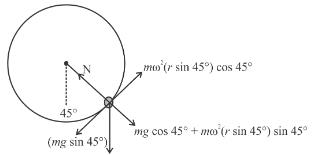For the bead not to slide upwards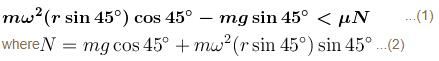From 1 and 2 we get,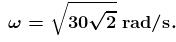*Answer can only contain numeric values
QUESTION: 10

A circular curve of a highway is designed for traffic moving at 72 km/h. If the radius of the curved path is 100m, the correct angle of banking of the road should be given by :n tan-1(n).  Find the value of nAnswer

Solution: